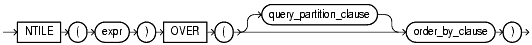## NTILE

#### Syntax

ntile::=Text description of ntile

#### Purpose

`NTILE` is an analytic function. It divides an ordered dataset into a number of buckets indicated by `expr` and assigns the appropriate bucket number to each row. The buckets are numbered 1 through `expr,` and `expr` must resolve to a positive constant for each partition.

The number of rows in the buckets can differ by at most 1. The remainder values (the remainder of number of rows divided by buckets) are distributed one for each bucket, starting with bucket 1.

If `expr` is greater than the number of rows, then a number of buckets equal to the number of rows will be filled, and the remaining buckets will be empty.

You cannot use `NTILE` or any other analytic function for `expr`. That is, you can use other built-in function expressions for `expr`, but you cannot nest analytic functions.

 See Also: "About SQL Expressions" for information on valid forms of `expr`

#### Examples

The following example divides into 4 buckets the values in the `salary` column of the `oe.employees` table from Department 100. The `salary` column has 6 values in this department, so the two extra values (the remainder of 6 / 4) are allocated to buckets 1 and 2, which therefore have one more value than buckets 3 or 4.

```SELECT last_name, salary, NTILE(4) OVER (ORDER BY salary DESC)
AS quartile FROM employees
WHERE department_id = 100;

LAST_NAME                     SALARY   QUARTILE
------------------------- ---------- ----------
Greenberg                      12000          1
Faviet                          9000          1
Chen                            8200          2
Urman                           7800          2
Sciarra                         7700          3
Popp                            6900          4
```